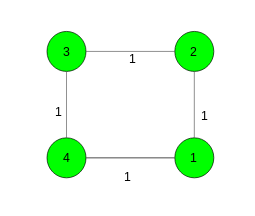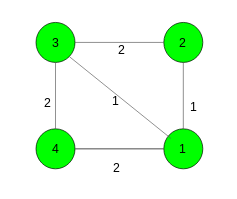# Find the weight of the minimum spanning tree

Given a connected undirected weighted graph with N nodes and M edges. The task is to perform given queries and find the weight of the minimum spanning tree. Queries are of three types:

1. query(1) -> Find the weight of the minimum spanning tree.
2. query(2, x, y) -> Change the weight of the edge between the nodes x and y to 0.
3. query(3, x, y) -> Restore the weight of the edge between the nodes x and y to its original weight.

Examples:

Input:query(2, 1, 2),
query(1),
query(3, 1, 2),
query(1)
Output:
2
3

Input:query(1),
query(2, 3, 4),
query(1)
Output :
4
2

## Recommended: Please try your approach on {IDE} first, before moving on to the solution.

Approach: Let’s first compute MST of the initial graph before performing any queries and let T be this MST. The crucial observation is that at any point while handling the queries, the weight of the MST of the current graph can be computed by running Kruskal’s algorithm on edges with zero weight at this point and edges of T. So, keep edges with weight zero in a data structure and after query of type 2 and type 3 compute weight of minimum spanning tree.

Below is the implementation of the above approach:

## C++

 `// C++ implementation of the approach ` `#include ` `using` `namespace` `std; ` `#define N 2005 ` ` `  `// To store vertices, edges ` `// and the required answer ` `int` `n, m, ans; ` ` `  `// To store parent and rank ` `int` `par[N], Rank[N]; ` ` `  `// To store edges and the edges in MST ` `vector > > edges, mst; ` ` `  `// To store the edges with weight zero ` `queue > zeros; ` ` `  `// Function for initialize ` `void` `initialize() ` `{ ` `    ``for` `(``int` `i = 0; i <= n; i++) { ` `        ``par[i] = i; ` `        ``Rank[i] = 0; ` `    ``} ` `} ` ` `  `// Function to add edges ` `void` `Add_edge(``int` `u, ``int` `v, ``int` `weight) ` `{ ` `    ``edges.push_back({ weight, { u, v } }); ` `} ` ` `  `// Utility function to find set of an element i ` `// (uses path compression technique) ` `int` `find(``int` `x) ` `{ ` `    ``if` `(par[x] != x) ` `        ``par[x] = find(par[x]); ` ` `  `    ``return` `par[x]; ` `} ` ` `  `// Function that performs union of two sets x and y ` `// (uses union by rank) ` `void` `Union(``int` `x, ``int` `y) ` `{ ` `    ``int` `xroot = find(x); ` `    ``int` `yroot = find(y); ` ` `  `    ``if` `(Rank[xroot] < Rank[yroot]) ` `        ``par[xroot] = yroot; ` `    ``else` `if` `(Rank[xroot] > Rank[yroot]) ` `        ``par[yroot] = xroot; ` `    ``else` `{ ` `        ``par[yroot] = xroot; ` `        ``Rank[xroot]++; ` `    ``} ` `} ` ` `  `// Function to compute minimum spanning tree ` `void` `compute_MST() ` `{ ` `    ``// Sort edges in increasing order of weight ` `    ``sort(edges.begin(), edges.end()); ` ` `  `    ``// Go through all the edges ` `    ``for` `(``int` `i = 0; i < m; i++) { ` `        ``int` `u = find(edges[i].second.first); ` `        ``int` `v = find(edges[i].second.second); ` ` `  `        ``if` `(u == v) ` `            ``continue``; ` ` `  `        ``// Build minimum spanning tree ` `        ``// and store minimum cost ` `        ``mst.push_back(edges[i]); ` `        ``ans += edges[i].first; ` `        ``Union(u, v); ` `    ``} ` `} ` ` `  `// Function to find the cost of minimum ` `// spanning tree ` `void` `Modified_Kruskal(pair<``int``, ``int``> x) ` `{ ` `    ``initialize(); ` ` `  `    ``// Make answer zero ` `    ``ans = 0; ` `    ``int` `sz = zeros.size(); ` ` `  `    ``// Keep the edges which only have zero weights ` `    ``// and remove all the other edges ` `    ``for` `(``int` `i = 0; i < sz; i++) { ` `        ``pair<``int``, ``int``> Front = zeros.front(); ` `        ``zeros.pop(); ` ` `  `        ``if` `(Front.first == x.first ` `            ``and Front.second == x.second) ` `            ``continue``; ` ` `  `        ``// Make union between the vertices of ` `        ``// edges which have weight zero and keep ` `        ``// them in queue ` `        ``Union(Front.first, Front.second); ` `        ``zeros.push(Front); ` `    ``} ` ` `  `    ``// Find the cost of the minimum spanning tree ` `    ``for` `(``int` `i = 0; i < mst.size(); i++) { ` `        ``int` `u = find(mst[i].second.first); ` `        ``int` `v = find(mst[i].second.second); ` ` `  `        ``if` `(u == v) ` `            ``continue``; ` ` `  `        ``ans += mst[i].first; ` `        ``Union(u, v); ` `    ``} ` `} ` ` `  `// Function to handle different queries ` `void` `query(``int` `type, ``int` `u = 0, ``int` `v = 0) ` `{ ` ` `  `    ``// Update edge weight to 0 ` `    ``if` `(type == 2) { ` `        ``// push edge in zeros ` `        ``zeros.push({ u, v }); ` `        ``Modified_Kruskal({ -1, -1 }); ` `    ``} ` ` `  `    ``// Restore edge weight to original value ` `    ``else` `if` `(type == 3) { ` `        ``// push edge in zeros ` `        ``zeros.push({ u, v }); ` `        ``Modified_Kruskal({ u, v }); ` `    ``} ` `    ``else` `        ``cout << ans << endl; ` `} ` ` `  `// Driver code ` `int` `main() ` `{ ` ` `  `    ``// Number of nodes and edges ` `    ``n = 4, m = 4; ` `    ``initialize(); ` ` `  `    ``// Add edges ` `    ``Add_edge(1, 2, 1); ` `    ``Add_edge(2, 3, 1); ` `    ``Add_edge(3, 4, 1); ` `    ``Add_edge(4, 1, 1); ` ` `  `    ``// Build the minimum spanning tree ` `    ``compute_MST(); ` ` `  `    ``// Execute queries ` `    ``query(2, 1, 2); ` `    ``query(1); ` `    ``query(3, 1, 2); ` `    ``query(1); ` ` `  `    ``return` `0; ` `} `

## Python3

 `# Python3 implementation of the approach ` `from` `collections ``import` `deque ` ` `  `N ``=` `2005` ` `  `# To store vertices, edges ` `# and the required answer ` `n, m, ans ``=` `0``, ``0``, ``0` ` `  `# To store parent and rank ` `par ``=` `[``0``] ``*` `N ` `Rank ``=` `[``0``] ``*` `N ` ` `  `# To store edges and the edges in MST ` `edges, mst ``=` `[], [] ` ` `  `# To store the edges with weight zero ` `zeroes ``=` `deque() ` ` `  `# Function for initialize ` `def` `initialize(): ` `    ``for` `i ``in` `range``(n ``+` `1``): ` `        ``par[i] ``=` `i ` `        ``Rank[i] ``=` `0` ` `  `# Function to add edges ` `def` `add_edge(u: ``int``, v: ``int``, weight: ``int``): ` `    ``edges.append((weight, (u, v))) ` ` `  `# Utility function to find set of an element i ` `# (uses path compression technique) ` `def` `find(x: ``int``) ``-``> ``int``: ` `    ``if` `par[x] !``=` `x: ` `        ``par[x] ``=` `find(par[x]) ` `    ``return` `par[x] ` ` `  `# Function that performs union of two sets x and y ` `# (uses union by rank) ` `def` `union(x: ``int``, y: ``int``): ` `    ``xroot ``=` `find(x) ` `    ``yroot ``=` `find(y) ` ` `  `    ``if` `Rank[xroot] < Rank[yroot]: ` `        ``par[xroot] ``=` `yroot ` `    ``elif` `Rank[xroot] > Rank[yroot]: ` `        ``par[yroot] ``=` `xroot ` `    ``else``: ` `        ``par[yroot] ``=` `xroot ` `        ``Rank[xroot] ``+``=` `1` ` `  `# Function to compute minimum spanning tree ` `def` `compute_MST(): ` `    ``global` `ans ` ` `  `    ``# Sort edges in increasing order of weight ` `    ``edges.sort() ` ` `  `    ``# Go through all the edges ` `    ``for` `i ``in` `range``(m): ` `        ``u ``=` `find(edges[i][``1``][``0``]) ` `        ``v ``=` `find(edges[i][``1``][``1``]) ` ` `  `        ``if` `u ``=``=` `v: ` `            ``continue` ` `  `        ``# Build minimum spanning tree ` `        ``# and store minimum cost ` `        ``mst.append(edges[i]) ` `        ``ans ``+``=` `edges[i][``0``] ` `        ``union(u, v) ` ` `  `# Function to find the cost of minimum ` `# spanning tree ` `def` `modified_kruskal(x): ` `    ``global` `ans ` `    ``initialize() ` ` `  `    ``# Make answer zero ` `    ``ans ``=` `0` `    ``sz ``=` `len``(zeroes) ` ` `  `    ``# Keep the edges which only have zero weights ` `    ``# and remove all the other edges ` `    ``for` `i ``in` `range``(sz): ` `        ``front ``=` `zeroes[``0``] ` `        ``zeroes.popleft() ` ` `  `        ``if` `front[``0``] ``=``=` `x[``0``] ``and` `front[``1``] ``=``=` `x[``1``]: ` `            ``continue` ` `  `        ``# Make union between the vertices of ` `        ``# edges which have weight zero and keep ` `        ``# them in queue ` `        ``union(front[``0``], front[``1``]) ` `        ``zeroes.append(front) ` ` `  `    ``# Find the cost of the minimum spanning tree ` `    ``for` `i ``in` `range``(``len``(mst)): ` `        ``u ``=` `find(mst[i][``1``][``0``]) ` `        ``v ``=` `find(mst[i][``1``][``1``]) ` ` `  `        ``if` `u ``=``=` `v: ` `            ``continue` `        ``ans ``+``=` `mst[i][``0``] ` `        ``union(u, v) ` ` `  `# Function to handle different queries ` `def` `query(``type``: ``int``, u``=``0``, v``=``0``): ` `    ``global` `ans ` ` `  `    ``# Update edge weight to 0 ` `    ``if` `type` `=``=` `2``: ` ` `  `        ``# push edge in zeros ` `        ``zeroes.append((u, v)) ` `        ``modified_kruskal((``-``1``, ``-``1``)) ` ` `  `    ``# Restore edge weight to original value ` `    ``elif` `type` `=``=` `3``: ` ` `  `        ``# push edge in zeros ` `        ``zeroes.append((u, v)) ` `        ``modified_kruskal((u, v)) ` `    ``else``: ` `        ``print``(ans) ` ` `  `# Driver Code ` `if` `__name__ ``=``=` `"__main__"``: ` ` `  `    ``# Number of nodes and edges ` `    ``n ``=` `4` `    ``m ``=` `4` `    ``initialize() ` ` `  `    ``# Add edges ` `    ``add_edge(``1``, ``2``, ``1``) ` `    ``add_edge(``2``, ``3``, ``1``) ` `    ``add_edge(``3``, ``4``, ``1``) ` `    ``add_edge(``4``, ``1``, ``1``) ` ` `  `    ``# Build the minimum spanning tree ` `    ``compute_MST() ` ` `  `    ``# Execute queries ` `    ``query(``2``, ``1``, ``2``) ` `    ``query(``1``) ` `    ``query(``3``, ``1``, ``2``) ` `    ``query(``1``) ` ` `  `# This code is contributed by ` `# sanjeev2552 `

Output:

```2
3
```My Personal Notes arrow_drop_upCheck out this Author's contributed articles.

If you like GeeksforGeeks and would like to contribute, you can also write an article using contribute.geeksforgeeks.org or mail your article to contribute@geeksforgeeks.org. See your article appearing on the GeeksforGeeks main page and help other Geeks.

Please Improve this article if you find anything incorrect by clicking on the "Improve Article" button below.

Improved By : sanjeev2552